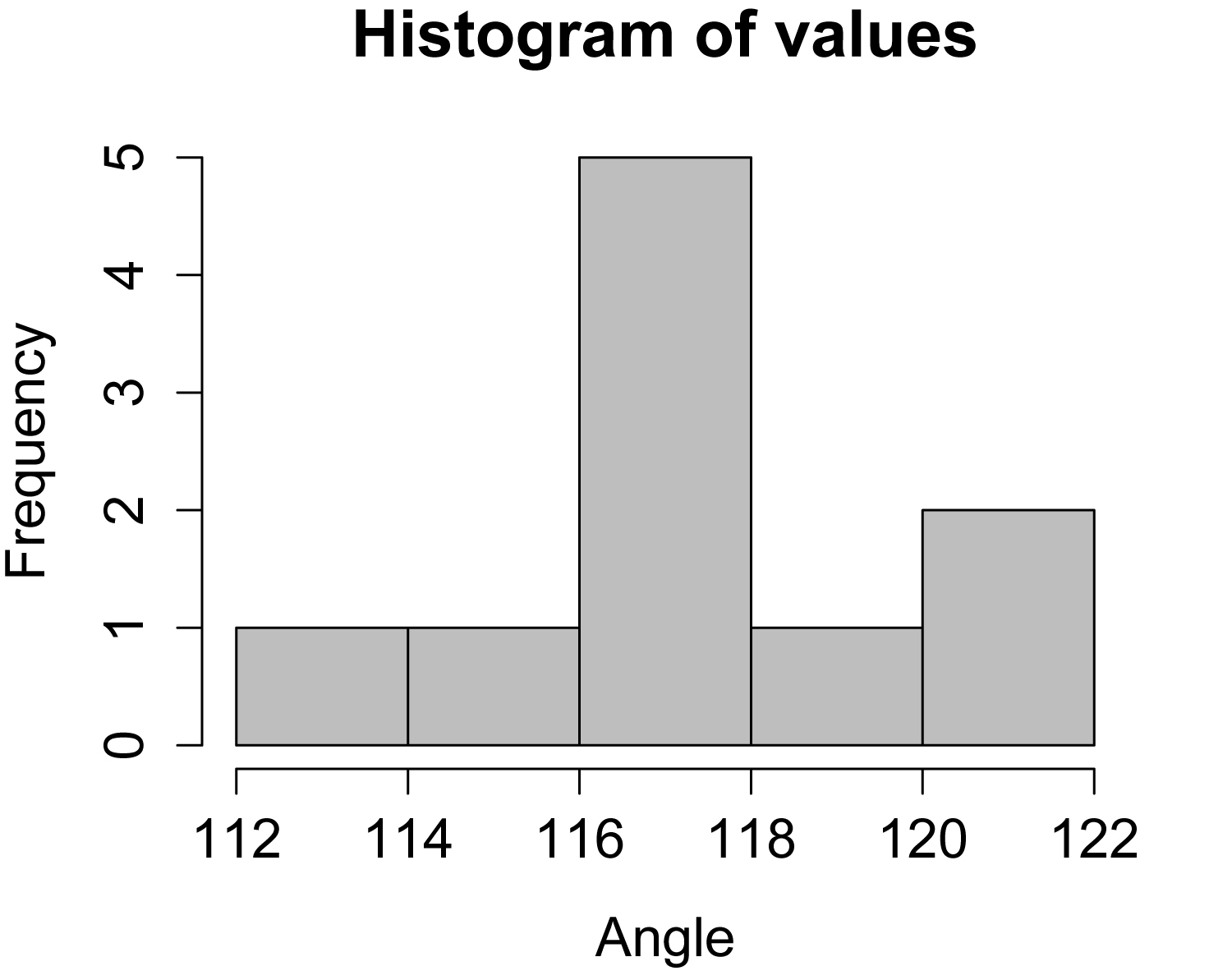## An R Companion for the Handbook of Biological Statistics

Salvatore S. Mangiafico

# Student’s t–test for One Sample

Introduction

When to use it

Null hypothesis

How the test works

Assumptions

See the Handbook for information on these topics.

### Example

#### One sample t-test with observations as vector

### --------------------------------------------------------------
### One-sample t-test, transferrin example, pp. 124
### --------------------------------------------------------------

observed    = c(0.52, 0.20, 0.59, 0.62, 0.60)
theoretical = 0

t.test(observed,
mu = theoretical,
conf.int = 0.95)

One Sample t-test

t = 6.4596, df = 4, p-value = 0.002958

#     #     #

Graphing the results

See the Handbook for information on this topic.

Similar tests

The paired t-test and two-sample t-test are presented elsewhere in this book.

### How to do the test

#### One sample t-test with observations in data frame

### --------------------------------------------------------------
### One-sample t-test, SAS example, pp. 125
### --------------------------------------------------------------

Input =("
Angle
120.6
116.4
117.2
118.1
114.1
116.9
113.3
121.1
116.9
117.0
")

observed    = Data\$ Angle
theoretical = 50

t.test(observed,
mu = theoretical,
conf.int=0.95)

One Sample t-test

t = 87.3166, df = 9, p-value = 1.718e-14

### Does not agree with Handbook. The Handbook results are incorrect.

### The SAS code produces the following result.

T-Tests

Variable      DF    t Value    Pr > |t|

angle          9      87.32      <.0001

#### Histogram

hist(Data\$ Angle,
col="gray",
main="Histogram of values",
xlab="Angle")Histogram of data in a single population from a one-sample t-test.  Distribution of these values should be approximately normal.

#     #     #

### Power analysis

#### Power analysis for one-sample t-test

### --------------------------------------------------------------
### Power analysis, t-test, one-sample,
###    hip joint example, pp. 125
126
### --------------------------------------------------------------

M1  = 70                        # Theoretical mean
M2  = 71                        # Mean to detect
S1  =  2.4                      # Standard deviation
S2  =  2.4                      # Standard deviation

Cohen.d = (M1 - M2)/sqrt(((S1^2) + (S2^2))/2)

library(pwr)

pwr.t.test(
n = NULL,                  # Observations
d = Cohen.d,
sig.level = 0.05,          # Type I probability
power = 0.90,              # 1 minus Type II probability
type = "one.sample",       # Change for one- or two-sample
alternative = "two.sided")

One-sample t test power calculation

n = 62.47518

#     #     #